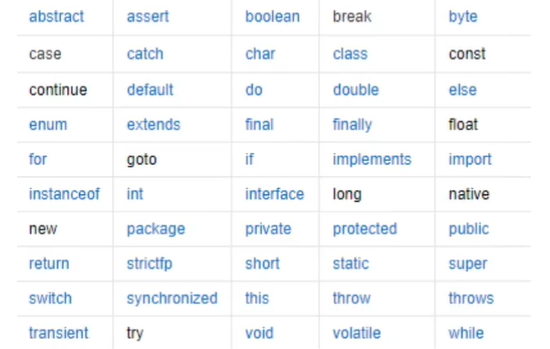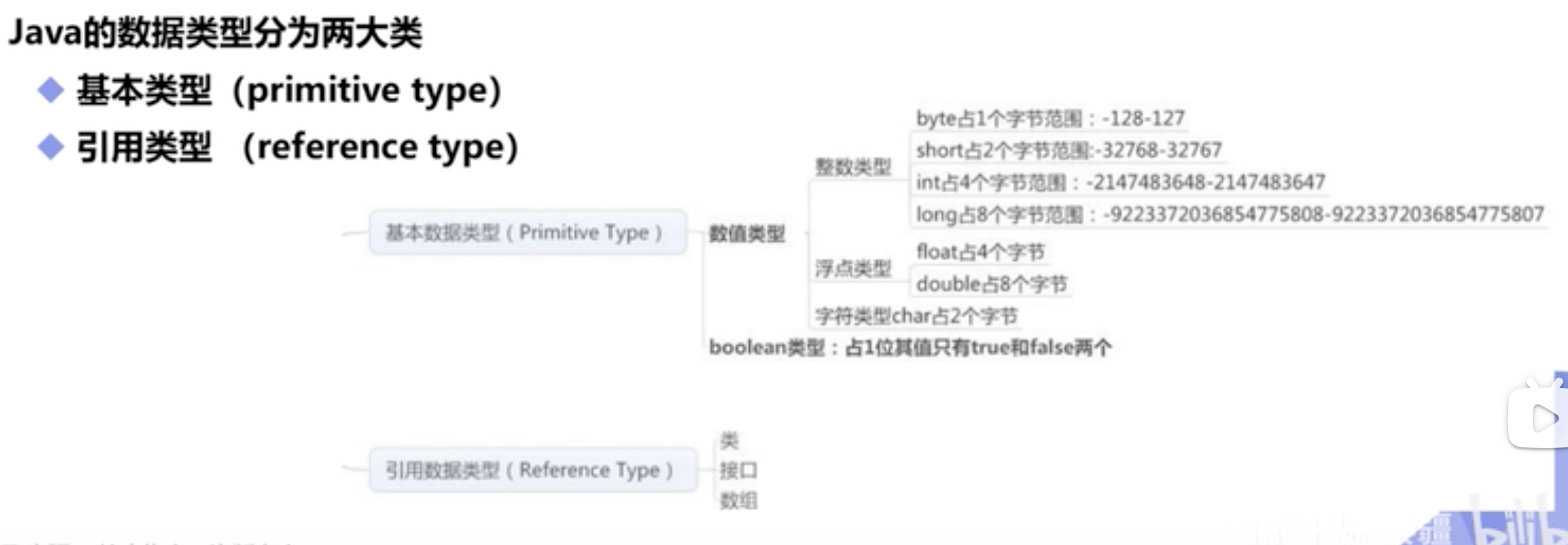# HelloWorld

``````public class HelloWorld{
public static void main(String[] args){
System.out.println("HelloWorld");
}
}
``````

# 注释

* 注释 */

``````public class HelloWorld {
/**
* 文档注释
* @param args
*/
public static void main(String[] args) {
// 单行注释
System.out.println("hello");
/*
多行注释
*/
}
}

``````

# 标识符和关键字# 数据类型

## 语言类型

java属于强类型语言``````//整数
int num1 = 10; //最常用，只要别超过21亿（2^31-1）
byte num2 = 20; //-128~127
short num3 = 30;
long num4 = 30L; //long类型数字后面要加个L(尽量用大写，小写l容易与1搞混)

//小数：浮点数
float num5 = 50.1F; //float类型数字后面要加个F
double num6 = 3.141592653589793238;

//字符
char name = '国';//字符只是单纯一个字
//字符串, String不是关键字，是类
//String namea = "薛之谦";

//布尔值：是非
boolean flag = true

``````

# 什么是字节

1B(byte) = 8bit，1字节等于8位

1bit表示1位

1Byte表示一个字节 1B=8b

1024B = 1KB, 1024KB = 1M, 1024M = 1G

# 数据类型扩展

``````//整数扩展： 二进制0b		八进制0		十进制		十六进制0x
int i = 10;
int i2 = 010; //八进制 8
int i3 = 0x10; //十六进制 16 0~9A~F 16
``````

## 浮点数扩展

``````//面试题：银行业务字母怎么表示钱? BigDecimal(大数,数学工具类)
//float double是有问题的，最好避免使用浮点数进行比较
float f = 0.1f; 	//0.1
double d = 1.0/10;  //0.1
System.out.println(f==d); //false
//**浮点数 位有限，舍入误差，大约**
//最好避免使用浮点数进行比较
float f1 = 23131313131f;
float f2 = f1+1;
System.out.println(f1==f2); //true,因为超过了精度
``````

## 字符扩展：所有字符本质还是数字

``````char c1 = ' a';
char c2 = '中';

System.out.println(c1);		//a
System.out.println((int)c1);//强制类型转换,97
System.out.println(c2);		//中
System.out.println((int)c2);//强制类型转换,20013
``````

## 编码

``````//Unicode表（97=a,65=A）2字节 0-65536
//U000~UFFFF 十六进制（u0061=a,相当于十进制的97）
System.out.println('a');  //a ''是转义字符
``````

## 布尔值扩展

``````boolean flag = true;
if(flag==true){} //新手
if(flag){}	//老手这样写 Less is More(代码要精简易读) 这两行代码是一样的
``````

# 类型转换

``````public  class Demo05{
public static void main(String[] args){
int i = 128;
byte b = (byte)i; //强制转换(类型)变量名 容量高到低强制
double d =i; //自动转换
System.out.println(i); //128
System.out.println(b); //-128 内存会溢出 -128~127(byte的最大值)
System.out.println(d); //128.0 低--高 自动转换
}
/*

1.不能对布尔值进行转换
2.不能把对象类型转换为不相干的类型
3.在把高容器转换到低容量的时候，强制转换,低到高,自动转换
4.转换可能存在内存溢出，或者精度问题
System.out.println((int)23.7); //23 丢失精度
*/
}
``````
``````char c = ‘a’;
int n = c+1;
System.out.println(n); //98 a为97
System.out.println((char)n); //b 把98转为字符
``````

## 当操作数比较大时，注意溢出问题

//JDK7新特性，数字之间可以用下划线分割

``````int money = 10_0000_0000; //10亿，下划线不会被打印出来
System.out.println(money); //1000000000

int years = 20;
int total = money*years; //数据大，溢出
System.out.println(total); //-1474836480

long total2 = money*years; //total在未被long转换前是是int，转换前就有溢出问题,所以用long转换没用
System.out.println(total2); //-1474836480

//解决方法
long total3 = money*(long)years; //先把一个数转Long
System.out.println(total3); //20000000000
————————————————

``````

# 变量

Java是一种强类型语言，每个变量都必须声明其类型

Java变量是程序中最基本的存储单元，要素包括变量名，变量类型和作用域

//数据类型 变量名 = 值; type varName [=value][{,varName[=value]}]; //可以使用逗号隔开同多个类型的变量，但不建议在一行定义多个变量 ————————————————

## 变量作用域

``````
public class Variable{
static int allClicks = 0; //类变量
String str = “hello world”; //实例变量

public void method(){
int i=0; //局部变量
}
}
————————————————
``````
``````public static void main(String[] args){
//属性:变量

//实例属性;从属于对象,如果不自行初始化,默认为0
//布尔型,默认是false
//除了基本类型,其余的都为null;

//类变量 static
static double salary =2500;

String name;
int age;

//main方法
public static void main(String[] args){

//局部变量:必须声明和初始化值,只在中间有用
int i = 10;
int i;//这是错误的没有初始化值
System.out.println(i);

//变量类型  变量名字 = new Demo08();
Demo08 demo08 = new Demo09();
System.out.println(demo08.age);//demo08.age调用了int age

//类变量 static
System.out.println(salary);调用类变量
}
//其他方法

}

}
``````

# 常量

//常量一般用大写字符
final 常量名=值;
//final代表常量 final double PI=3.14;

//修饰符 不存在先后顺序，static可以写final后面 static final doube PI=3.14; //类变量，该类下的全局范围

``````public class Demo09{

//修饰符 不存在先后顺序，static可以写final后面
static final doube PI=3.14; //类变量，该类下的全局范围

}
``````

————————————————